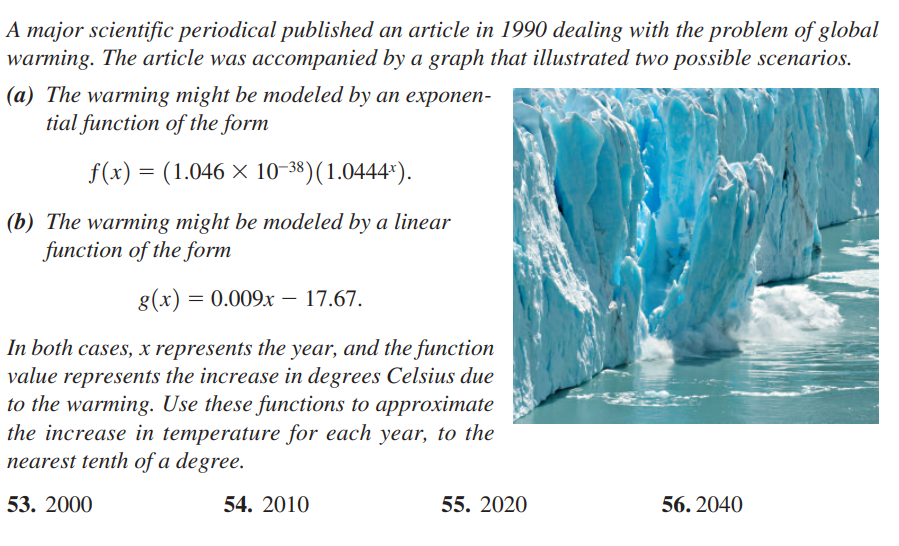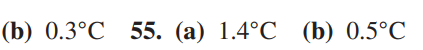### ¿Todavía tienes preguntas de matemáticas?

Pregunte a nuestros tutores expertos
Algebra
PreguntaA major scientific periodical published an article in $$1990$$ dealing with the problem of global warming. The article was accompanied by a graph that illustrated two possible scenarios.

(a) The warming might be modeled by an exponen- tial function of the form $$f ( x ) = ( 1.046 \times 10 ^ { - 38 } ) ( 1.0444 ^ { x } )$$ .

(b) The warming might be modeled by a linear function of the form $$g ( x ) = 0.009 x - 17.67$$

In both cases, $$x$$ represents the year, and the function value represents the increase in degrees Celsius due to the warming. Use these functions to approximate the increase in temperature for each year, to the nearest tenth of a degree.

53. $$2000$$  54. $$2010 \quad$$ 55. $$2020 \quad$$ 56. $$2040$$